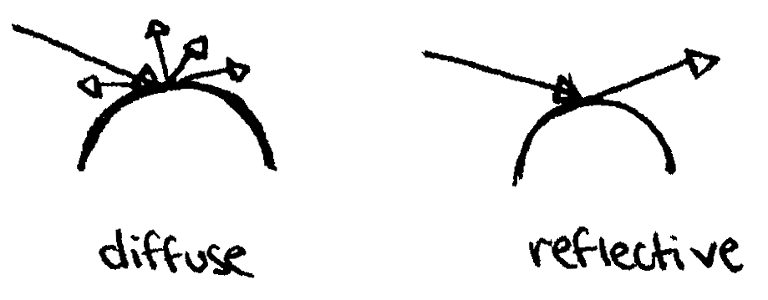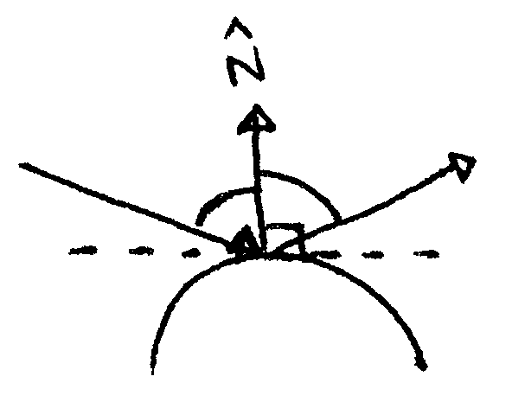# Build your own 3D renderer - Recursive ray tracing and reflections

Light bounces of all geometry, but different materials bounce light in different ways. Some materials are highly diffuse, meaning they scatter bounced light in many directions, sending a little bit of light in each direction. In contrast, some materials are highly reflective, meaning they send bounced light in one direction. Many materials are a combination of the two.A diffuse surface scatters light in many directions, while a reflective surface concentrates light in one direction.

The angle of the reflected light depends on the angle of the incoming light, that is the angle of incidence. We compute the reflection direction just like we did when computing the specular component in the Phong illumination model, but this time based on the view direction: hat bbR = 2(hat bbN * hat bbV) hat bbN - hat bbV. Here, hat bbV is just the direction of the ray we cast, but normalized. To see the derivation of this, refer back to the section on the Phong illumination model.The reflection angle is the same as the angle of incidence.

To find what would be reflected back to the viewer, we cast a ray starting at the viewed point on the geometry, going in the reflected direction vec bbR. This is the basis of recursive ray tracing, where the usual ray tracing algorithm is applied to compute the reflection. This can cause further reflections, so we must limit the recursion depth—corresponding to the number of light bounces simulated—to some constant.

In the Phong illumination model, the ambient term approximates light left over after many bounces, and the specular term approximates the reflection of a light source sending light directly to an object. Thus, these terms do not change due to reflection. Similarly, the diffuse term is still calculated the same way for a mostly reflective object, but its contribution should be diminished to account for the fact that only a small portion of the incoming light is scattered. This is done by reducing k_d, the diffuse constant, for the the object.

Finally, we add in one more term to the Phong illumination model: the reflected light weighted by a reflectivity constant k_r. Usually, the larger k_r is, the lower k_d is, and vice versa.

Recursive ray tracing is also the basis of simulating refraction, where light passes through a translucent object. While we will not implement refraction rays in our ray tracer, the general principle is mostly the same as the reflectance rays, differing only in the direction the refraction ray is cast.